# Denoising by Sobolev and Total Variation Regularization¶

Important: Please read the installation page for details about how to install the toolboxes. $\newcommand{\dotp}{\langle #1, #2 \rangle}$ $\newcommand{\enscond}{\lbrace #1, #2 \rbrace}$ $\newcommand{\pd}{ \frac{ \partial #1}{\partial #2} }$ $\newcommand{\umin}{\underset{#1}{\min}\;}$ $\newcommand{\umax}{\underset{#1}{\max}\;}$ $\newcommand{\umin}{\underset{#1}{\min}\;}$ $\newcommand{\uargmin}{\underset{#1}{argmin}\;}$ $\newcommand{\norm}{\|#1\|}$ $\newcommand{\abs}{\left|#1\right|}$ $\newcommand{\choice}{ \left\{ \begin{array}{l} #1 \end{array} \right. }$ $\newcommand{\pa}{\left(#1\right)}$ $\newcommand{\diag}{{diag}\left( #1 \right)}$ $\newcommand{\qandq}{\quad\text{and}\quad}$ $\newcommand{\qwhereq}{\quad\text{where}\quad}$ $\newcommand{\qifq}{ \quad \text{if} \quad }$ $\newcommand{\qarrq}{ \quad \Longrightarrow \quad }$ $\newcommand{\ZZ}{\mathbb{Z}}$ $\newcommand{\CC}{\mathbb{C}}$ $\newcommand{\RR}{\mathbb{R}}$ $\newcommand{\EE}{\mathbb{E}}$ $\newcommand{\Zz}{\mathcal{Z}}$ $\newcommand{\Ww}{\mathcal{W}}$ $\newcommand{\Vv}{\mathcal{V}}$ $\newcommand{\Nn}{\mathcal{N}}$ $\newcommand{\NN}{\mathcal{N}}$ $\newcommand{\Hh}{\mathcal{H}}$ $\newcommand{\Bb}{\mathcal{B}}$ $\newcommand{\Ee}{\mathcal{E}}$ $\newcommand{\Cc}{\mathcal{C}}$ $\newcommand{\Gg}{\mathcal{G}}$ $\newcommand{\Ss}{\mathcal{S}}$ $\newcommand{\Pp}{\mathcal{P}}$ $\newcommand{\Ff}{\mathcal{F}}$ $\newcommand{\Xx}{\mathcal{X}}$ $\newcommand{\Mm}{\mathcal{M}}$ $\newcommand{\Ii}{\mathcal{I}}$ $\newcommand{\Dd}{\mathcal{D}}$ $\newcommand{\Ll}{\mathcal{L}}$ $\newcommand{\Tt}{\mathcal{T}}$ $\newcommand{\si}{\sigma}$ $\newcommand{\al}{\alpha}$ $\newcommand{\la}{\lambda}$ $\newcommand{\ga}{\gamma}$ $\newcommand{\Ga}{\Gamma}$ $\newcommand{\La}{\Lambda}$ $\newcommand{\si}{\sigma}$ $\newcommand{\Si}{\Sigma}$ $\newcommand{\be}{\beta}$ $\newcommand{\de}{\delta}$ $\newcommand{\De}{\Delta}$ $\newcommand{\phi}{\varphi}$ $\newcommand{\th}{\theta}$ $\newcommand{\om}{\omega}$ $\newcommand{\Om}{\Omega}$

This numerical tour explores the use of variational minimization to perform denoising. It consider the Sobolev and the Total Variation regularization functional (priors).

In :
warning off
warning on


## Prior and Regularization¶

For a given image $f$, a prior $J(f) \in \mathbb{R}$ assign a score supposed to be small for the image of interest.

A denoising of some noisy image $y$ is obtained by a variational minimization that mixes a fit to the data (usually using an $L^2$ norm) and the prior. $$\min_f \frac{1}{2}\|y-f\|^2 + \lambda J(f)$$

If $J(f)$ is a convex function of $f$, then the minimum exists and is unique.

The parameter $\tau>0$ should be adapted to the noise level. Increasing its value means a more agressive denoising.

If $J(f)$ is a smooth functional of the image $f$, a minimizer of this problem can be computed by gradient descent. It defines a series of images $f^{(\ell)}$ indexed by $\ell \in \mathbb{N}$ as $$f^{(\ell+1)} = f^{(\ell)} + \tau \left( f^{(\ell)}-y + \lambda \text{Grad}J(f^{(\ell)}) \right).$$

Note that for $f^{(\ell)}$ to converge with $\ell \rightarrow +\infty$ toward a solution of the problem, $\tau$ needs to be small enough, more precisely, if the functional $J$ is twice differentiable, $$\tau < \frac{2}{1 + \lambda \max_f \|D^2 J(f)\|}.$$

## Sobolev Prior¶

The Sobolev image prior is a quadratic prior, i.e. an Hilbert (pseudo)-norm.

First we load a clean image.

In :
n = 256;
name = 'hibiscus';
f0 = rescale( sum(f0,3) );


For a smooth continuous function $f$ it is defined as $$J(f) = \int \|\nabla f(x)\|^2 d x$$

Where the gradient vector at point $x$ is defined as $$\nabla f(x) = \left( \frac{\partial f(x)}{\partial x_1},\frac{\partial f(x)}{\partial x_2} \right)$$

For a discrete pixelized image $f \in \mathbb{R}^N$ where $N=n \times n$ is the number of pixel, $\nabla f(x) \in \mathbb{R}^2$ is computed using finite difference.

In :
Gr = grad(f0);


One can compute the norm of gradient, $d(x) = \|\nabla f(x)\|$.

In :
d = sqrt(sum3(Gr.^2,3));


Display.

In :
clf;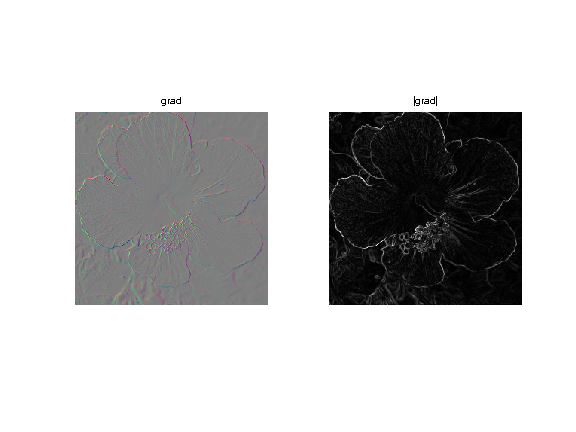The Sobolev norm is the (squared) $L^2$ norm of $\nabla f \in \mathbb{R}^{N \times 2}$.

In :
sob = sum(d(:).^2);


## Heat Regularization for Denoising¶

Heat regularization smoothes the image using a low pass filter. Increasing the value of |\lambda| increases the amount of smoothing.

Add some noise to the original image.

In :
sigma = .1;
y = f0 + randn(n,n)*sigma;


The solution $f^{\star}$ of the Sobolev regularization can be computed exactly using the Fourier transform. $$\hat f^{\star}(\omega) = \frac{\hat y(\omega)}{ 1+\lambda S(\omega) } \quad\text{where}\quad S(\omega)=\|\omega\|^2.$$

This shows that $f^{\star}$ is a filtering of $y$.

Useful for later: Fourier transform of the observations.

In :
yF = fft2(y);


Compute the Sobolev prior penalty |S| (rescale to [0,1]).

In :
x = [0:n/2-1, -n/2:-1];
[Y,X] = meshgrid(x,x);
S = (X.^2 + Y.^2)*(2/n)^2;


Regularization parameter:

In :
lambda = 20;


Perform the denoising by filtering.

In :
fSob = real( ifft2( yF ./ ( 1 + lambda*S) ) );


Display.

In :
clf;
imageplot(clamp(fSob));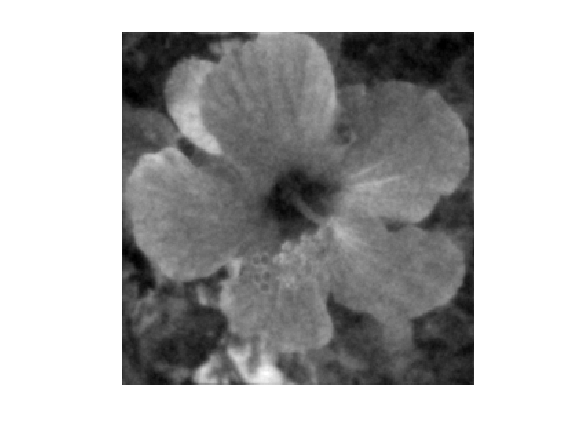Exercise 1

Compute the solution for several value of $\lambda$ and choose the optimal |lambda| and the corresponding optimal denoising |fSob0|. You can increase progressively lambda and reduce considerably the number of iterations. lot

In :
exo1()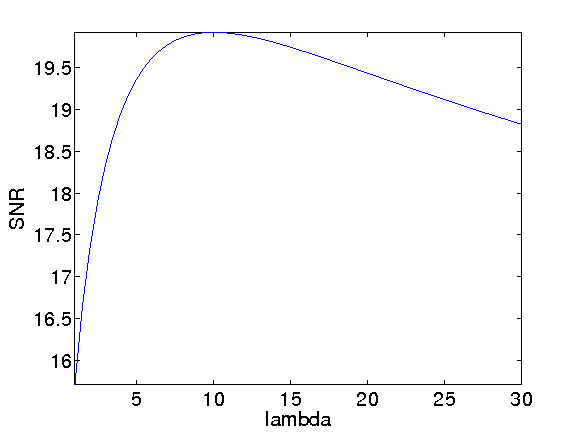In :
%% Insert your code here.


Display best "oracle" denoising result.

In :
esob = snr(f0,fSob0);  enoisy = snr(f0,y);
clf;
imageplot(clamp(y), strcat(['Noisy ' num2str(enoisy,3) 'dB']), 1,2,1);
imageplot(clamp(fSob0), strcat(['Sobolev regularization ' num2str(esob,3) 'dB']), 1,2,2);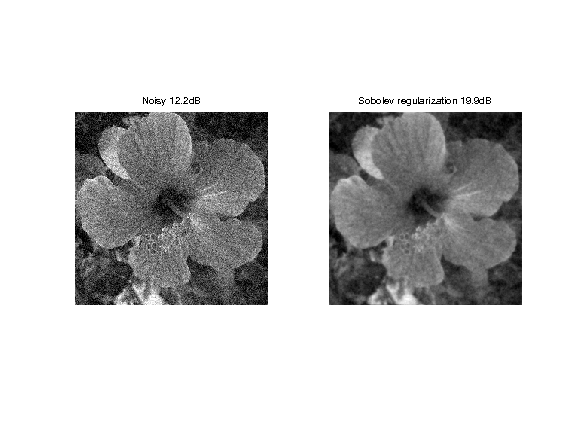## Total Variation Prior¶

The total variation is a Banach norm. On the contrary to the Sobolev norm, it is able to take into account step edges.

The total variation of a smooth image $f$ is defined as $$J(f)=\int \|\nabla f(x)\| d x$$

It is extended to non-smooth images having step discontinuities.

The total variation of an image is also equal to the total length of its level sets. $$J(f)=\int_{-\infty}^{+\infty} L( S_t(f) ) dt$$

Where $S_t(f)$ is the level set at $t$ of the image $f$ $$S_t(f)=\{ x \backslash f(x)=t \}$$

Exercise 2

Compute the total variation of |f0|. isplay

In :
exo2()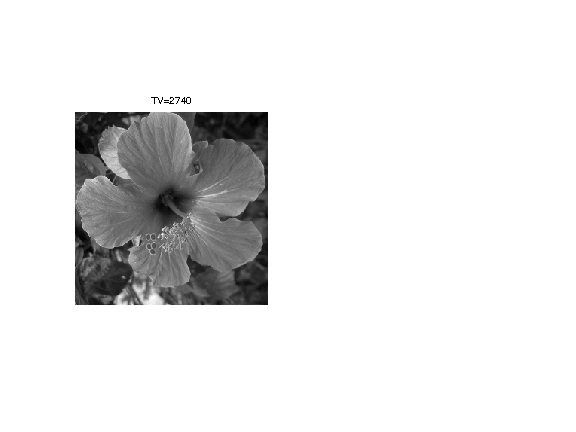In :
%% Insert your code here.


The Gradient of the TV norm is $$\text{Grad}J(f) = \text{div}\left( \frac{\nabla f}{\|\nabla f\|} \right) .$$

The gradient of the TV norm is not defined if at a pixel $x$ one has $\nabla f(x)=0$. This means that the TV norm is difficult to minimize, and its gradient flow is not well defined.

To define a gradient flow, we consider instead a smooth TV norm $$J_\epsilon(f) = \int \sqrt{ \varepsilon^2 + \| \nabla f(x) \|^2 } d x$$

This corresponds to replacing $\|u\|$ by $\sqrt{\varepsilon^2 + \|u\|^2}$ which is a smooth function.

We display (in 1D) the smoothing of the absolute value.

In :
u = linspace(-5,5)';
clf;
subplot(2,1,1); hold('on');
plot(u, abs(u), 'b');
plot(u, sqrt(.5^2+u.^2), 'r');
title('\epsilon=1/2'); axis('square');
subplot(2,1,2); hold('on');
plot(u, abs(u), 'b');
plot(u, sqrt(1^2+u.^2), 'r');
title('\epsilon=1'); axis('square');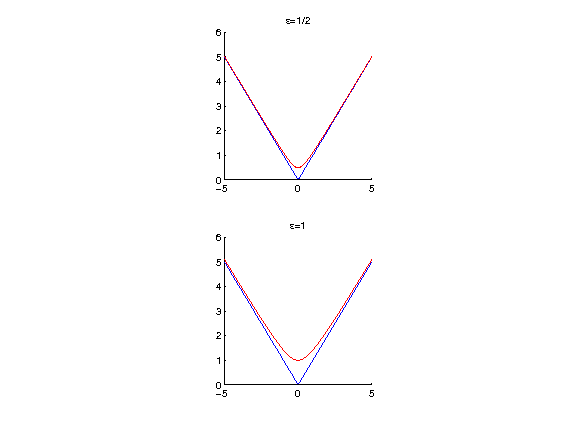The Gradient of the smoothed TV norm is $$\text{Grad}J(f) = \text{div}\left( \frac{\nabla f}{\sqrt{\varepsilon^2 + \|\nabla f\|^2}} \right) .$$

When $\varepsilon$ increases, the smoothed TV gradient approaches the Laplacian (normalized by $1/\varepsilon$).

In :
epsilon_list = [1e-9 1e-2 1e-1 .5];
clf;
for i=1:length(epsilon_list)
G = div( Gr ./ repmat( sqrt( epsilon_list(i)^2 + d.^2 ) , [1 1 2]) );
imageplot(G, strcat(['epsilon=' num2str(epsilon_list(i))]), 2,2,i);
end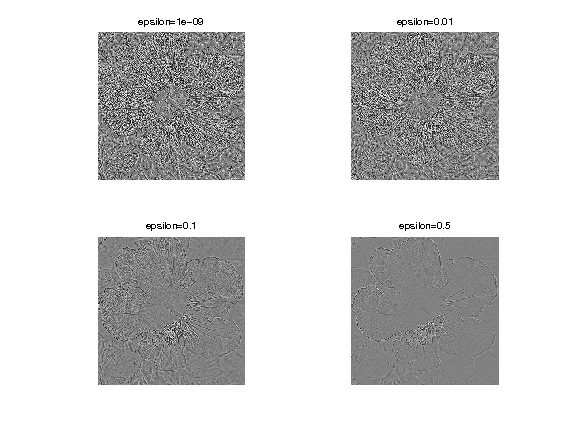## Total Variation Regulariation for Denoising¶

Total variation regularization was introduced by Rudin, Osher and Fatemi, to better respect the edge of image than linear filtering.

We set a small enough regularization parameter.

In :
epsilon = 1e-2;


Define the regularization parameter $\lambda$.

In :
lambda = .1;


The step size for diffusion should satisfy: $$\tau < \frac{2}{1 + \lambda 8 / \varepsilon} .$$

In :
tau = 2 / ( 1 + lambda * 8 / epsilon);


Initialization of the minimization.

In :
fTV = y;


Compute the gradient of the smoothed TV norm.

In :
Gr = grad(fTV);
d = sqrt(sum3(Gr.^2,3));
G = -div( Gr ./ repmat( sqrt( epsilon^2 + d.^2 ) , [1 1 2]) );


One step of descent.

In :
fTV = fTV - tau*( y-fTV + lambda* G);


Exercise 3

Compute the gradient descent and monitor the minimized energy.

In :
exo3()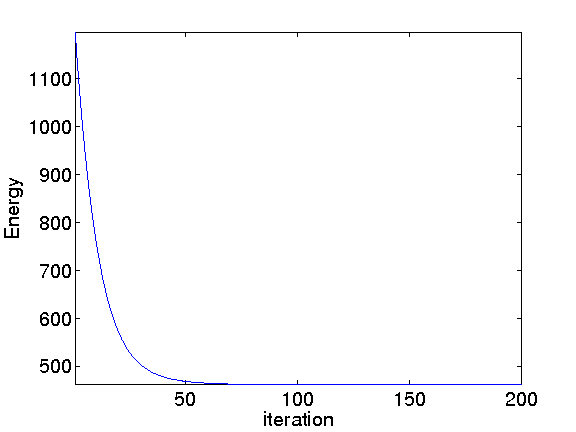In :
%% Insert your code here.


Display the image.

In :
clf;
imageplot(fTV);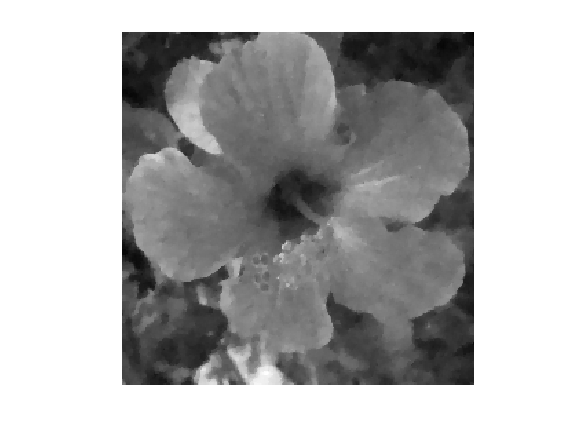Exercise 4

Compute the solution for several value of $\lambda$ and choose the optimal $\lambda$ and the corresponding optimal denoising |fSob0|. You can increase progressively $\lambda$ and reduce considerably the number of iterations.

In :
exo4()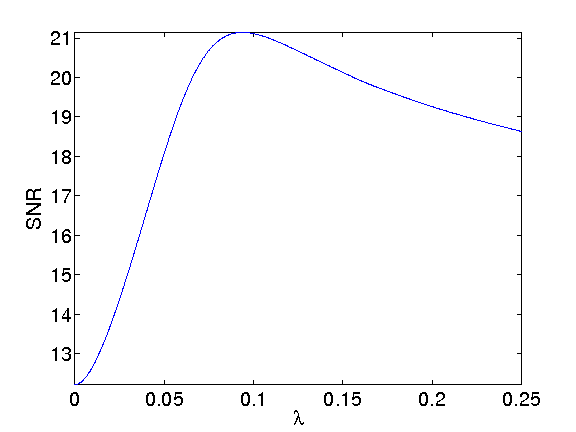In :
%% Insert your code here.


Display best "oracle" denoising result.

In :
etvr = snr(f0,fTV0);
clf;
imageplot(clamp(y), strcat(['Noisy ' num2str(enoisy,3) 'dB']), 1,2,1);
imageplot(clamp(fTV0), strcat(['TV regularization ' num2str(etvr,3) 'dB']), 1,2,2);